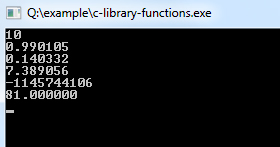# C Library Functions

The C library functions are provided by the system and stored in the library. The C library function is also called an inbuilt function in C programming.

To use Inbuilt Function in C, you must include their respective header files, which contain prototypes and data definitions of the function.

## C Program to Demonstrate the Use of Library Functions

Example:
``````#include<stdio.h>
#include<ctype.h>
#include<math.h>

void main()
{
int i = -10, e = 2, d = 10; /* Variables Defining and Assign values */  float rad = 1.43;
double d1 = 3.0, d2 = 4.0;

printf("%d\n", abs(i));